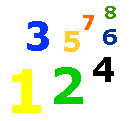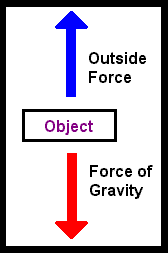# 4-11 Concept of Force

 Force is the exertion of a push or pull on an object.  The force of gravity pulls on an object on Earth.  Lift a book from your desk.  The force of gravity pulls on the book as you lift.  The force in your arms is greater than the force of gravity, thus the book moves upward.  As you put the book down, you can feel the force of gravity pull on the book.  If you hold the book in your hands, the force of gravity and the force of your arms is equal.  The book stays in one place.The amount of force needed to lift an object depends on the object's weight.  Force can be expressed in a unit of mass such as the pound.  Scientist use a special unit, called a Newton, to measure forces.  A Newton is about .225 pounds.  It would take about 4 Newtons to hold up a one pound object.  Other forces are electrical and magnetic.  All these forces can be measured in pounds.  Complete the questions below. Force is the push or on an object. Scientist measure force with the . To lift a glass, the force in your arm must be than the force of gravity. When you hold an object steady in your arms, the force of gravity and the force in your arms is . Two forces other than gravity are electrical and . A two pound object would measure about newtons.